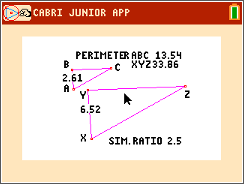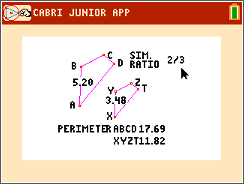••• ##### Device
• TI-84 Plus
• TI-84 Plus Silver Edition
•TI-84 Plus C Silver Edition
•TI-84 Plus CE
• ##### Software

TI Connect™ CE

# Geometry: Ratios of Similar Figures

by Texas Instruments#### Overview

Students will explore the ratio of perimeter, area, surface area, and volume of similar figures in two-dimensional figures.

#### Key Steps

•Students will use the Cabri™ Jr. app to discover the ratios of similar figures. In Problem 1, they will explore the ratios of the perimeters and areas of similar triangles.

•In Problem 2, students will explore the ratios of the perimeters and areas of similar quadrilaterals.